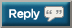# Thread: Let's OHP 2,019,000 pounds in 2019!

1. Just read the OP. How are you counting dumbbell presses; 70lb = 70lbs or 140lbs? ...The total weight that goes overhead.2.The total weight that goes overhead.
... minus the weight of your arms.3. Barbell OHP (strict)
+3,740 = 150,345
Press in Snatch:
10x20kg

Military Press:
5x52.5kg
3x55kg
3x57.5kg
2x72.5kg (Figured out it wasn't 62.5kg, LOL)
5x65kg

1,045kg ~= 2,305lbs

-----

150,345lbs + 2,305lbs = 152,350lbs4. +
Strict Press [45x10 + 95x5 + 135x2 + 155x4x4] = 3,675

=156,0255. +
Strict Press [45x10 + 95x5 + 135x2 + 155x4x4] = 3,675

=156,025
Checking in for 1st time. Running Fierce 5 Intermediate so only doing OHP once per week, but anyway:

1/10/19 - 2805
1/17/19 - 2540
1/24/19 - 2870
TOTAL 8215

156,025 + 8215 = 164,2406.The total weight that goes overhead.
... minus the weight of your arms.lol, thanks

Checking in for 1st time. Running Fierce 5 Intermediate so only doing OHP once per week, but anyway:

1/10/19 - 2805
1/17/19 - 2540
1/24/19 - 2870
TOTAL 8215

156,025 + 8215 = 164,240
2825 deload session last night + 4700 dumbbells last week that I didn't count = 7,525 + 164,240 = 171,765lbs7. + 3,860 (not counting arm weight) = 175,6258. Push Presses this morning
42kg x 5
42kg x 5
42kg x 5
42kg x 5
42kg x 5

=2310lbs

=177,935#9. Push Presses this morning
42kg x 5
42kg x 5
42kg x 5
42kg x 5
42kg x 5

=2310lbs

=177,935#
Press in Snatch:
10x20kg

5x55kg
5x55kg
5x57.5kg
5x60kg
5x60kg

-----

=177,935 + 3,610 = 181,54510. +3,740 lbs (barbell strict OHP, working sets)
= 185,28511. Snatch Grip Push presses

20kg x 5
35kg x 5
42kg 5x5

=2915lbs

=188,20012. Snatch Grip Push presses

20kg x 5
35kg x 5
42kg 5x5

=2915lbs

=188,200
From last evening workout

40 kg x 5
50 kg x 5
55 kg x 5
61 kg x 5
61 kg x 5
61 kg x 3

= 3345 lbs

= 191,545 lbs13. From last evening workout

40 kg x 5
50 kg x 5
55 kg x 5
61 kg x 5
61 kg x 5
61 kg x 3

= 3345 lbs

= 191,545 lbs
+ 6,175 = 197,72014. Press in Snatch:
4x8x20kg

Get it there for Overhead Squats:
1x35kg
1x42.5kg
1x47.5kg
1x55kg
1x60kg

880kg

-----

+1,940 = 199,66015. Press in Snatch:
4x8x20kg

Get it there for Overhead Squats:
1x35kg
1x42.5kg
1x47.5kg
1x55kg
1x60kg

880kg

-----

+1,940 = 199,660
+ 4,770 = 204,43016. 45x10
65x8
95x6
135x5
150x5
180x7
190x4
200x1
210x1

5,395 + 204,430 = 209,82517. 209,825

+ 45x10 + 95x5 + 135x2 + (145x6)x4 = 4,675

= 214,500 lbs18. 209,825

+ 45x10 + 95x5 + 135x2 + (145x6)x4 = 4,675

= 214,500 lbs
+ 4,720 = 219,22019. Push Presses
20kg x 5
30kg x 5
43kg x 5
43kg x 5
43kg x 5
43kg x 5
43kg x 5

=2415lbs

=221,635 lbs20. Just found this thread. I added up all the OHP weight from my logs for the year so far and converted to pounds.... 32,355lbs so far. Trying to up it this year.
Here's my log link if anyone wants to give me tips or critique https://forum.bodybuilding.com/showt...post1564297061

221,635+32,355=253,99021. Snatch Grip Push Presses
20kg x 5
36kg x 5
44kg 5 x 5

=3036lbs

253,990+ 3036= 257,02622. Snatch Grip Push Presses
20kg x 5
36kg x 5
44kg 5 x 5

=3036lbs

253,990+ 3036= 257,026
+4,355 = 261,38123. Yesterday workout +3,465 barbell strict OHP
=264,84624. Just some seated dumbbell work so far this week. Lower back is not cooperating atm.

2050 + 264,846 = 266,89625. Just some seated dumbbell work so far this week. Lower back is not cooperating atm.

2050 + 264,846 = 266,896
+4,510 = 271,40626. disastrous abandoned workout exhausted total power failure... nothing was working. However I did some of the press I planned (barbell strict OHP) before I abandoned and retreated to the showers, so here's a small contribution. +2501
=273,90627. Strict standing barbell press. 45x10 + 95x5 + 120x3 + 135x3 + 155x8 + (100x10)x5 = 7,937

+273,906

= 281,83628. Just found this thread, I'll go through my logs for earlier this year, but this week was 4,725#.

281,836 + 4,725 = 286,56129. Just found this thread, I'll go through my logs for earlier this year, but this week was 4,725#.

281,836 + 4,725 = 286,561
+ 2,050 = 288,61130. Thu 1/31 - 2655
Thu 2/7 - 3000
Total 5655

+5655 = 294,266#### Posting Permissions

• You may not post new threads
• You may not post replies
• You may not post attachments
• You may not edit your posts